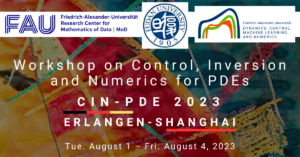# Control of Advection-Diffusion Equations on Networks and Singular Limits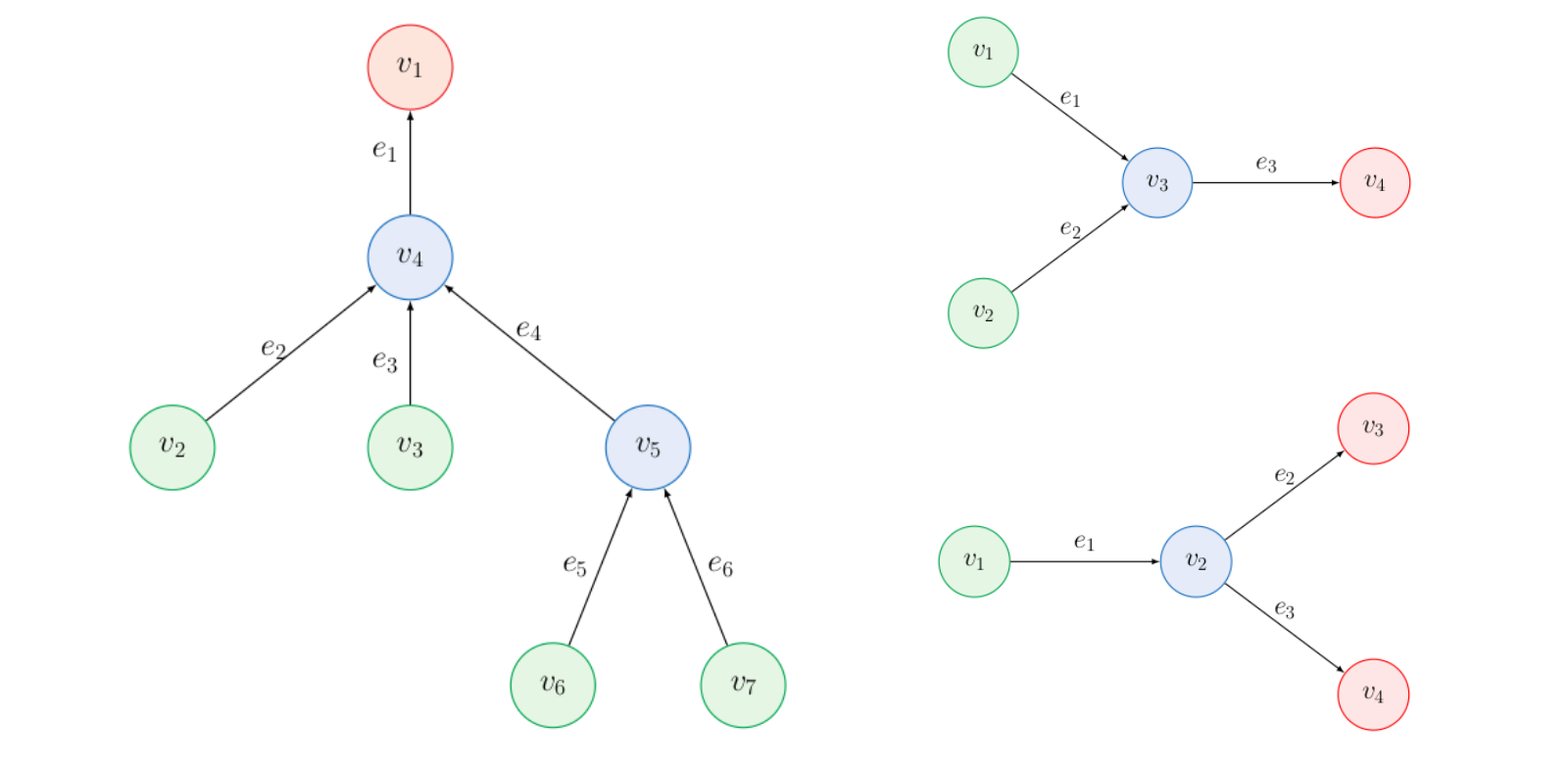## Control of Advection-Diffusion Equations on Networks and Singular Limits

By Jon Asier Bárcena-Petisco, Márcio Cavalcante, Giuseppe Maria Coclite, Nicola de Nitti and Enrique Zuazua

Introduction
In the past few decades, models based on partial differential equations have been very effective in tackling many problems dealing with flows on networks (e.g. irrigation channels, gas pipelines, blood circulation, vehicular traffic, supply chains, air traffic management — see  for a survey of the topic).

In particular, linear advection-diffusion equations on graphs have been employed to describe the flow of a fluid with a dissolved contaminant through a network of one-dimensional cracks (see ). Following , we represent the network by a finite, directed, and connected graph \mathcal G = (\mathcal V, \mathcal E) with vertices \mathcal V =\{v_1,\dots,v_n\} and edges \mathcal E =\{e_1,\dots,e_m\}\subset \mathcal V \times \mathcal V . For every edge e \in \mathcal E , we define an incidence vector (n^e)_{v \in \mathcal V} by
n^e(v) = -1 if e = (v,\cdot), \quad n^e(v) = 1 if e=(\cdot, v), \quad and \quad n^e(v) =0 otherwise.

Here, n^e plays the same role as the normal vector for problems in multi-dimensional domains.

For any v\in \mathcal V, we define the set of incident edges \mathcal E(v):=\{e\in \mathcal E:n^{e}(v)\neq 0\} and distinguish between inner vertices \mathcal V_0 := \{ v \in \mathcal V : |\mathcal E(v)| \geq 2 \} and boundary or external vertices \mathcal V_{\partial}:=\mathcal V\backslash\mathcal V_0.
We suppose that, for all v\in\cal V_0, there are e_1,e_2\in\cal E(v) such that n^{e_1}(v)=-1 and n^{e_2}(v)=1.

We may model the proposed problem with the system (1):
\begin{cases} a^e\partial_t y_\epsilon^e + b^e \partial_x y_\epsilon^e -\epsilon\partial^2_{xx}y_\epsilon^e =0, &\text{ in } (0,T)\times e, \ e\in\cal E, \\ y_\epsilon^e(t,v)=u^v_\epsilon(t), &\text{ on } (0,T)\times \cal V_{\partial}, \\ y_\epsilon^{e_1}(t,v) = y^{e_2}_\epsilon(t,v), & t\in(0,T), \ v\in\cal V, \forall e_1,e_2\in\cal E(v), \\ \sum _{e\in \cal E(v)}(b^e y_\epsilon^e-\epsilon\partial_x y^e_\epsilon)n^e=0, &\text{ on } (0,T)\times \cal V_{0},\\ y_\epsilon(0,\cdot)=y_0, &\text{ on } \cal E, \end{cases}

with a^e,b^e,\epsilon>0 and for a fixed time-horizon T>0. Each edge is identified with a closed interval [x_e,\overset{\wedge}{x}_e], and the left end (resp. right end) is identified with the vertex v such that n^e(v)=-1 (resp. n^e(v)=1). We shall also use the notation y_\epsilon := (y^{e_1}_\epsilon, \dots, y^{e_m}_\epsilon) and u = (u^{v_1}_\epsilon, \dots, u^{v_k}_\epsilon), where k = |V_\partial| and m=|\mathcal{E}|. The boundary condition (1)_3 is a continuity condition in the internal nodes and (1)_4 implies that the flux of the mass is null. Here, y_\epsilon^e denotes the concentration of the contaminant and b^e the flow rate in each edge of the graph and u^e_\epsilon is the boundary datum on each boundary vertex of the graph, which we may use as control. We additionally assume (2):

which is a balance relation for the flow across a junction. In addition to being an assumption used in the parabolic energy estimate to annihilate the terms at junctions, condition (2) is motivated by the corresponding hyperbolic problem, for which it ensures that energy does not increase at junctions. Such condition is also pivotal in proving the vanishing viscosity approximation result in .

In our paper , we study the appropriate boundary data (boundary control) in order to lead the solution of the parabolic problem (1) to rest; and, in particular, its behavior as the diffusivity parameter \epsilon vanishes. In other words, we are interested in controlling the solution of (1) across the network and in studying the cost of controllability as \epsilon \to 0^+, i.e. as the solution of (1) approaches the one of the hyperbolic problem

\begin{cases} a^e\partial_t y^e + b^e \partial_x y^e =0,& \text{ in } (0,T)\times e, \ e\in \cal E, \\ y^e(t,v)=u^v(t), & \text{ on } (0,T)\times \cal V_{\partial}^{in},\\ y^{e_1}(t,v) = y^{e_2}(t,v), &\ t\in(0,T), \ v\in\cal V, \ \forall e_1,e_2\in\cal E^{out}(v),\\ \sum _{e\in \cal E^{in}(v)}b^ey^e= y^{e_1}(t,v)\sum_{e\in\cal E^{out}(v)}b^e, & \text{ on } (0,T)\times \cal V_{0}, \ e_1 \in \mathcal E^{out}(v),\\ y(0,\cdot)=y_0, & \text{ on } \cal E, \end{cases}

where the contaminant does not undergo diffusion and is only driven by the (constant) velocity of the liquid flow. Here, \cal V^{in}_\partial are the vertices from which the flow is coming into the network (i.e. with just outgoing edges), \cal V^{out}_\partial are the vertices where the flow goes out of the network (i.e. with just incoming edges), \cal E^{in}(v) are the edges incoming into v, and \cal E^{out}(v) are the edges outgoing from v (see Figure 1). The junction condition (3)_3 expresses continuity of the concentrations exiting a junction and (3)_4 gives the conservation of the mass.
Throughout the paper, we mainly consider tree-shaped networks (that is, networks without loops), an example of which is given in Figure 1.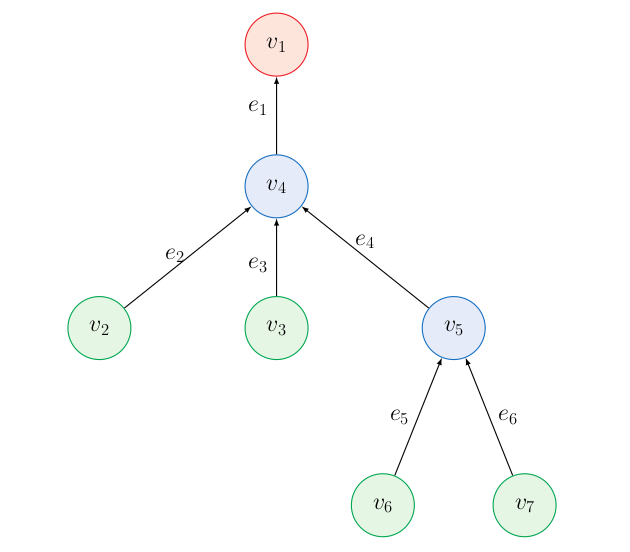Figure 1. An example of tree with edges e_1=(v_4,v_1), e_2=(v_2,v_4), e_3=(v_3,v_4), and e_4 = (v_5,v_4), e_5 = (v_6,v_5), e_6 = (v_7,v_5); inner vertices V_{0}=\{v_4,v_5\} (blue), and boundary vertices V_{\partial}=\{v_1,v_2,v_3, v_6,v_7\}. We split the set of boundary vertices into inflow and outflow vertices: V_{\partial}^{in}=\{v_2,v_3,v_6,v_7\} (green) and V_\partial^{out}=\{v_1\} (red), respectively. The set \cal E (v_4)=\{e_1,e_2,e_3,e_4\} denotes the edges adjacent to the junction v_4. In a similar manner, we can split the set \cal E(v_4) by \cal E^{in}(v_4)=\{e_2,e_3,e_4\} and \cal E^{out}(v_4)=\{e_1\} into edges that go into or out of the vertex v_4. The arrows illustrate the flow direction. The non-zero incidence vectors are
n^{e_1}(v_1) = n^{e_2}(v_4) = n^{e_3}(v_4) = n^{e_4}(v_4) = n^{e_5}(v_5) = n^{e_6}(v_5) = 1 and n^{e_1}(v_4) = n^{e_2}(v_4) = n^{e_3}(v_4) = n^{e_4}(v_5) = n^{e_5}(v_6) = n^{e_6}(v_7) = - 1.

The Transport Equation
Our first result concerns the null controllability of problem (3) on a tree. In particular, we are interested in controlling the flow across the network by means of controls placed on the inflow vertices V^{in}_{\partial}.

To this end, we define an upper bound and a lower bound on the time in which the information propagates across the network (i.e., the maximal and minimal travel time of the characteristics across the network).

Definition 1 (Propagation time on a network). Let G =(V, E) be a tree. We define the functions \overset{\wedge}{T}, \overset{\sim}{T}: \mathcal V \to \R_+ as follows:
\begin{aligned} \overset{\wedge}{T}(v)=0,\quad \overset{\sim}{T}(v)=0 && \text{ if } v\in\cal V_{\partial}^{in}, \\ \overset{\wedge}{T}(v)= \sup_{e=(v^e,v)\in \cal E^{in}(v)}\left( \overset{\wedge}{T}(v^e)+ \frac{a^el^e}{b^e}\right) && \text{ if } v\in\cal V_0\cup \cal V_\partial^{out},\\ \overset{\sim}{T}(v)= \inf_{e=(v^e,v)\in \cal E^{in}(v)}\left( \overset{\sim}{T}(v^e)+ \frac{a^el^e}{b^e}\right) && \text{ if } v\in\cal V_0\cup \cal V_\partial^{out}. \end{aligned} The times \overset{\wedge}{T}(v) and \overset{\sim}{T}(v) are respectively the maximal and minimal propagation time required for information to reach v \in V from a node in V_{\partial}^{in}.

Since the network \cal G has no loops (being a tree), we can prove inductively that \overset{\wedge}{T} and \overset{\sim}{T} are well-defined.

Example 1 (Propagation times). Let consider the graph in Figure 2, made of vertices v_1, v_2, v_3, v_4 and edges e_1=[v_1,v_3]\simeq[0,2], e_2=[v_2,v_3]\simeq [0,1], and e_3=[v_3,v_4]\simeq [0,2]. We consider the equation (3) with a^{e_1}=a^{e_2}=a^{e_3}=b^{e_1}=b^{e_2}=1 and b^{e_3}=2. We can compute the maximal travel time to reach v \in \mathcal V as follows:

\overset{\wedge}{T}(v_1) = \overset{\wedge}{T}(v_2) = 0, \quad \overset{\wedge}{T}(v_3) = \sup_{i=1,2} \big(\overset{\wedge}{T}(v_i) + l^i\big) = \max \{ 1, 2\} = 2, \quad \overset{\wedge}{T}(v_4) = \overset{\wedge}{T}(v_3) + \tfrac{l^3}{b^3} = 2 + 1 = 3.

Moreover, we compute the minimal travel time to reach v\in\cal V as follows:
\overset{\sim}{T}(v_1) = \overset{\sim}{T}(v_2) = 0, \quad \overset{\sim}{T}(v_3) = \inf_{i=1,2} \big(\overset{\sim}{T}(v_i) + l^i\big) = \min \{ 1, 2\} = 1, \quad \overset{\sim}{T}(v_4) = \overset{\sim}{T}(v_3) + \tfrac{l^3}{b^3} = 1 + 1 = 2.Figure 2. Star-graph with edges e_1=(v_1,v_3), e_2=(v_2,v_3), e_3=(v_3,v_4); inner vertices V_0=\{v_3\} (blue), and boundary vertices V_\partial=\{v_1,v_2,v_4\}. We split the set of boundary vertices into inflow and outflow vertices: V_{\partial}^{in}=\{v_1,v_2\} (green) and V_\partial^{out}=\{v_4\} (red), respectively.

By relying on this notion of propagation time and on the classical method of characteristics, we can prove the following controllability result with an inductive argument.

Theorem 1 (Null-controllability for the hyperbolic problem). Let G=(V, E) be a tree and let y be the solution of (3) for u=0. Then, y=0 for all T\geq \max_{v\in \cal V_\partial^{out}}\overset{\wedge}{T}(v).

In particular, we prove by induction a stronger result: y^e(T,x)=0 for all x\in e=[v_1,v_2]\simeq [0,l^e] and
T\geq \overset{\wedge}{T}(v_1)+ \frac{a^ex}{b^e}.

Moreover, the following result regarding the minimal propagation time holds.

Proposition 1 (Minimal time for the null-controllability for the hyperbolic problem). Let \mathcal G = (\mathcal V, \mathcal E) be a tree. Then, system (3) is not null-controllable for T< \max_{v\in \cal V_\partial^{out}}\overset{\sim}{T}(v).

Proposition 1 is also proved by the method of characteristics and induction. As shown in , the optimal time for null-controllability can be almost any in [\max_{v\in \cal V_\partial^{out}}\overset{\sim}{T}(v), \max_{v\in \cal V_\partial^{out}}\overset{\wedge}{T}(v)] (by possibly using non-zero controls) by considering the right network, so the upper and lower bound are optimal if we do not add any extra hypothesis on the structure of the network.

Control and Singular Limit
Our final main theorem provides estimates on the cost of controllability as \epsilon \to 0^+ for the parabolic problem (1).

We consider the following quantity which measures the cost of the null-controllability of (1):
\begin{aligned} K(\cal E,T,a^e,b^e, G,\overset{\wedge}{V}_\partial) := \sup_{y_0 \in L^2(E) \setminus \{0\}} \inf_{u \in U} \frac{|| u ||_{L^2(0,T)}}{|| y_0 ||_{L^2(E)}}, \end{aligned} where U:= U(y_0,\cal E, T, a^e,b^e, G) denotes the subset of \{u:\ell^2(V_\partial):u(v)=0\ \forall v\in V_\partial\setminus \overset{\wedge}{V}_\partial\} such that the solution of (1) satisfies y(T,\cdot)=0.

Regarding the cost of controllability, from the hyperbolic result in Theorem 1, we expect the following behavior: for small times, K \to + \infty as \cal E \to 0^+; on the other hand, for T sufficiently large, K \to 0 as \cal E \to 0^+. We prove a quantitative estimate on these limits.

Theorem 2 (Estimates on the cost of controllability). Let \mathcal G = (\mathcal V, \mathcal E) be a tree and assume that (2) holds.
(1) There exists c >0 such that, for \varepsilon small enough and all T< \max_{v\in \cal V_\partial^{out}}\overset{\sim}{T}(v), the following lower bound holds:
K(\varepsilon, T, a^e,b^e,\mathcal G, \cal V^{in}_{\partial}) \ge c e^{c / \varepsilon}.

(2) There exist \overline{T}, c >0 such that, for \varepsilon \text{ small enough and all } T < \bar{T} the following lower bound holds:
K(\varepsilon, T, a^e,b^e,\mathcal G, V_{\partial}) \ge c e^{c/ \varepsilon}.

(3) There exist T_0,c,C >0 \text{ such that, for } \varepsilon \text{ small enough and all } T \ge T_0 the following upper bound holds:
K(\varepsilon, T, a^e,b^e,\mathcal G, \cal V_\partial) \le C e^{-c/\varepsilon}.

The proof of Theorem 2 is based on the H.U.M. (see, for example, ) — that is, on study the cost of observation of the adjoint variable.
The adjoint system is given by
\begin{cases} -a^e\partial_t \varphi_\varepsilon^e - b^e \partial_x \varphi_\varepsilon^e -\varepsilon\partial^2_{xx}\varphi_\varepsilon^e =0,& \text{ in } (0,T)\times e, e\in\cal E,\\ \varphi_\varepsilon^e(v)=0, & \text{ on }(0,T)\times \cal V_{\partial},\\ \varphi_\varepsilon^{e_1}(t,v) = \varphi^{e_2}_\varepsilon(t,v), &\ t\in(0,T), \ v\in\cal V, \ \forall e_1,e_2\in\cal E^{out}(v),\\ \sum_{e\in \cal E(v)}\varepsilon\partial_{n^e} \varphi^e_\varepsilon(t,v)=0, & \text{ on } (0,T)\times \cal V_{0}, \\ \varphi_\varepsilon(T,\cdot)=\varphi_T, & \text{ on } \cal E, \end{cases} where we have used (2) to obtain (4) and (8). We recall that, by using the H.U.M., the cost of the control defined in (4) is equivalently given by
K(\cal E,T,a^e,b^e,\mathcal G,\overset{\wedge}{\mathcal V}_\partial) = \sup_{\varphi^T \in L^2(\cal E)\setminus\{0\}} \frac{|| \varphi(0,\cdot)||_{L^2(\cal E)}}{\left(\iint_{(0,T)\times \overset{\wedge}{\cal V}_\partial} |\partial_\nu \varphi|^2 dt \right)^{1/2}}.

Roughly speaking, the strategy of our proofs is as follows:

• for Claims (1) and (2), we obtain that the cost explodes for small times by considering data for the adjoint problem supported far away from the observation domains and computing its observability cost — this explodes as \varepsilon \to 0^+ because for small times the mass remains in the domain, but the mass reaching the observation domain is of order \exp(C\varepsilon^{-1});
• for Claim (3), we show that the cost decays for large times by using first the decay of the free solutions, and then, when the mass of the state is almost null, by exactly observing the mass.

The result of Theorem 2 is quite sharp. We note that, in general, system (1) cannot be controlled for any \varepsilon>0 by acting on fewer than |\mathcal V_{\partial}| -1 vertices.

Proposition 2 (Lack of null-controllability for general graphs). Let G=(V, E) be the graph in Figure 3, made of the vertices v_1,v_2,v_3,v_4 and the edges e_1=[v_1,v_2]\simeq[0,1], e_2=[v_2,v_3]\simeq [0,1], and e_3=[v_2,v_4]\simeq [0,1]. Then, system (1) (with coefficients b^{e_1}=a^{e_1}=a^{e_2}=a^{e_3}=1 and b^{e_2}=b^{e_3}=\frac{1}{2}) is not approximately controllable by acting only on v_1 (i.e. if u^{v_3}=u^{v_4}=0) for any \varepsilon>0.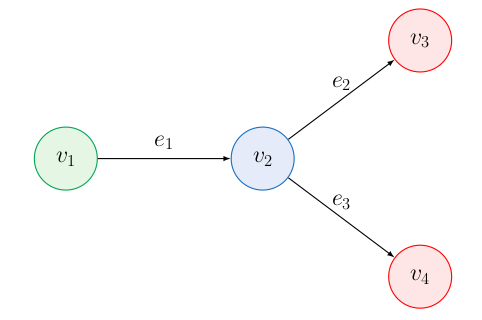Figure 3. Star-graph with edges e_1=(v_1,v_2), e_2=(v_2,v_3), e_3=(v_2,v_4); inner vertices V_0=\{v_2\} (blue), and boundary vertices V_\partial=\{v_1,v_3,v_4\}. We split the set of boundary vertices into inflow and outflow vertices: V_{\partial}^{in}=\{v_1\} (green) and V_\partial^{out}=\{v_3,v_4\} (red), respectively.

Heuristically, the reason is that, by symmetry, the effect of the control on e_2 and e_3 is identical, so we cannot control both y^{e_2} and y^{e_3} simultaneously. The proof is made rigorous by a duality argument.

#### References

 A. Bressan, S. Canic, M. Garavello, M. Herty, and B. Piccoli. Flows on networks: recent results and perspectives. EMS Surv. Math. Sci., 1(1):47–111, 2014
 J. A. Bárcena-Petisco, M. Cavalcante, G. M. Coclite, N. De Nitti, and E. Zuazua. Control of hyperbolic and parabolic equations on networks and singular limits. Preprint, 2021
 H. Egger and T. Kugler. Damped wave systems on networks: exponential stability and uniform approximations. Numer. Math., 138(4):839–867, 2018
 H. Egger and N. Philippi. On the transport limit of singularly perturbed convection–diffusion problems on networks. Mathematical Methods in the Applied Sciences, 44:5005–5020, 2021
 J.-L. Lions. Contrôlabilité exacte, perturbations et stabilisation de systèmes distribués. Tome 1. Contrôlabilité exacte. [Exact controllability]. With appendices by E. Zuazua, C. Bardos, G. Lebeau and J. Rauch, volume 8 of Recherches en Mathématiques Appliquées [Research in Applied Mathematics]. Masson, Paris, 1988.
 S. F. Oppenheimer. A convection-diffusion problem in a network. Appl. Math. Comput., 112(2-3):223–240, 2000

### You might like!#### FAU MoD Lecture: Free boundary regularity for the obstacle problem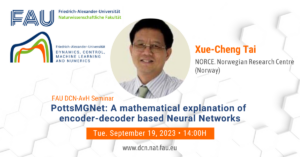#### PottsMGNet: A Mathematical Explanation of Encoder-Decoder Based Neural Networks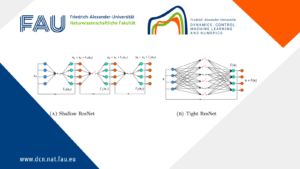#### Neural ODEs for interpolation and transport: From tight to shallow